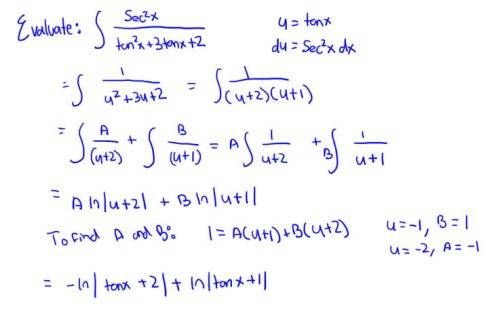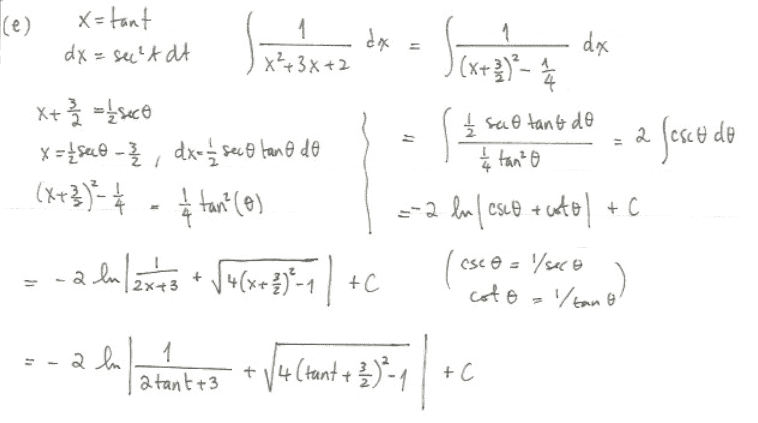# Partial Fraction Decomposition problem

## Homework Statement

Evaluate ∫((secx)^2)/[((tanx)^2)+(3tanx)+2]

## Homework Equations

Partial fraction decomposition

## The Attempt at a Solution

So here's what I did:But this is incorrect. It says the correct answer is -2lnabs($\frac{1}{2tanx+3}$+$\sqrt{4(tanx+3/2)^{2}-1}$), which was achieved by making the same u-substitution, completing the square, and then doing a trig substitution. I'm not sure why my method is incorrect. Any help would be great, thanks!

haruspex
Homework Helper
Gold Member
2020 Award
I agree with your answer (and method!). Seems to me that the other answer is a decreasing function in the range 0 to 1, yet from the integral it should clearly be increasing. If you post all the working of the other method I'll see if I can find the error.

•1 person
Ohh okay, I'm glad it's not incorrect then! Here's the other solution I was referring to :haruspex
Homework Helper
Gold Member
2020 Award
There's an error here: csc = 1/sec.

•1 person
There's an error here: csc = 1/sec.
Ohh okay I see. Thanks so much for the help! Would you be willing to help me with another partial fraction decomposition question?

haruspex